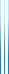# PublicationsManuscripts
## Orthogonal matrices with optimal $L_2$ norm
Question 8 in Chapter 2 of The Probabilistic Method asks us to show that for every $n\times n$ orthogonal matrix and $1\leq k\leq n$, there is a column such that the squared $L_2$ norm of its first $k$ entries is at least $k/n$, or at most $k/n$. It also asks for an example in which this is tight. We exhibit such an example which works simultaneously for all $k$.